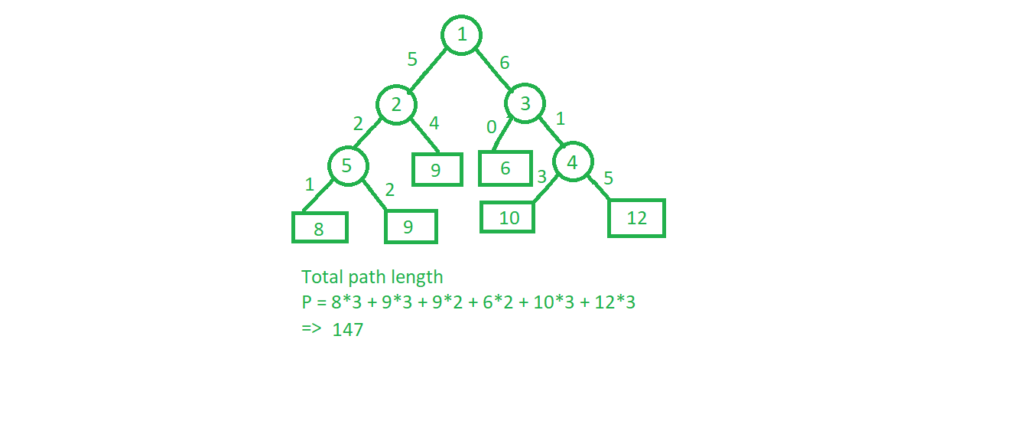# Extended Binary Tree

Extended binary tree is a type of binary tree in which all the null sub tree of the original tree are replaced with special nodes called external nodes whereas other nodes are called internal nodesHere the circles represent the internal nodes and the boxes represent the external nodes.

Properties of External binary tree

1. The nodes from the original tree are internal nodes and the special nodes are external nodes.
2. All external nodes are leaf nodes and the internal nodes are non-leaf nodes.
3. Every internal node has exactly two children and every external node is a leaf. It displays the result which is a complete binary tree

Below is an example of making an extended binary tree in C++ by making all the external nodes as ‘-1’

## C++

 `// C++ program to make an extended binary tree ` `#include ` `using` `namespace` `std; ` ` `  `// A Tree node ` `struct` `Node { ` `    ``int` `key; ` `    ``struct` `Node *left, *right; ` `}; ` ` `  `// Utility function to ` `// create a new node ` `Node* newNode(``int` `key) ` `{ ` `    ``Node* temp = ``new` `Node; ` `    ``temp->key = key; ` `    ``temp->left = temp->right = NULL; ` `    ``return` `(temp); ` `} ` ` `  `// Function for inorder traversal ` `void` `traverse(Node* root) ` `{ ` `    ``if` `(root != NULL) { ` `        ``traverse(root->left); ` `        ``cout << root->key << ``" "``; ` `        ``traverse(root->right); ` `    ``} ` `    ``else` `{ ` ` `  `        ``// Making external nodes ` `        ``root = newNode(-1); ` `        ``cout << root->key << ``" "``; ` `    ``} ` `} ` ` `  `// Driver code ` `int` `main() ` `{ ` `    ``Node* root = newNode(1); ` `    ``root->left = newNode(2); ` `    ``root->right = newNode(3); ` `    ``root->left->left = newNode(5); ` `    ``root->right->right = newNode(4); ` ` `  `    ``traverse(root); ` ` `  `    ``return` `0; ` `} `

## Java

 `// Java program to make an extended binary tree ` `class` `GFG ` `{ ` `     `  `// A Tree node ` `static` `class` `Node  ` `{ ` `    ``int` `key; ` `    ``Node left, right; ` `}; ` ` `  `// Utility function to create a new node ` `static` `Node newNode(``int` `key) ` `{ ` `    ``Node temp = ``new` `Node(); ` `    ``temp.key = key; ` `    ``temp.left = temp.right = ``null``; ` `    ``return` `(temp); ` `} ` ` `  `// Function for inorder traversal ` `static` `void` `traverse(Node root) ` `{ ` `    ``if` `(root != ``null``)  ` `    ``{ ` `        ``traverse(root.left); ` `        ``System.out.print(root.key + ``" "``); ` `        ``traverse(root.right); ` `    ``} ` `    ``else` `    ``{ ` ` `  `        ``// Making external nodes ` `        ``root = newNode(-``1``); ` `        ``System.out.print(root.key + ``" "``); ` `    ``} ` `} ` ` `  `// Driver code ` `public` `static` `void` `main(String args[]) ` `{ ` `    ``Node root = newNode(``1``); ` `    ``root.left = newNode(``2``); ` `    ``root.right = newNode(``3``); ` `    ``root.left.left = newNode(``5``); ` `    ``root.right.right = newNode(``4``); ` ` `  `    ``traverse(root); ` `} ` `} ` ` `  `// This code is contributed by Arnab Kundu `

## C#

 `// C# program to make an extended binary tree  ` `using` `System; ` ` `  `class` `GFG  ` `{  ` `         `  `    ``// A Tree node  ` `    ``public` `class` `Node  ` `    ``{  ` `        ``public` `int` `key;  ` `        ``public` `Node left, right;  ` `    ``};  ` `     `  `    ``// Utility function to create a new node  ` `    ``static` `Node newNode(``int` `key)  ` `    ``{  ` `        ``Node temp = ``new` `Node();  ` `        ``temp.key = key;  ` `        ``temp.left = temp.right = ``null``;  ` `        ``return` `(temp);  ` `    ``}  ` `     `  `    ``// Function for inorder traversal  ` `    ``static` `void` `traverse(Node root)  ` `    ``{  ` `        ``if` `(root != ``null``)  ` `        ``{  ` `            ``traverse(root.left);  ` `            ``Console.Write(root.key + ``" "``);  ` `            ``traverse(root.right);  ` `        ``}  ` `        ``else` `        ``{  ` `     `  `            ``// Making external nodes  ` `            ``root = newNode(-1);  ` `            ``Console.Write(root.key + ``" "``);  ` `        ``}  ` `    ``}  ` `     `  `    ``// Driver code  ` `    ``public` `static` `void` `Main()  ` `    ``{  ` `        ``Node root = newNode(1);  ` `        ``root.left = newNode(2);  ` `        ``root.right = newNode(3);  ` `        ``root.left.left = newNode(5);  ` `        ``root.right.right = newNode(4);  ` `     `  `        ``traverse(root);  ` `    ``}  ` `} ` ` `  `// This code is contributed by AnkitRai01  `

Output:

```-1 5 -1 2 -1 1 -1 3 -1 4 -1
```

Application of extended binary tree:

1. Calculate weighted path length: It is used to calculate total path length in case of weighted tree.Here, the sum of total weights is already calculated and stored in the external nodes and thus makes it very easier to calculate the total path length of a tree with given weights. The same technique can be used to update routing tables in a network.
2. To convert binary tree in Complete binary tree: The above-given tree having removed all the external nodes, is not a complete binary tree. To introduce any tree as complete tree, external nodes are added onto it. Heap is a great example of a complete binary tree and thus each binary tree can be expressed as heap if external nodes are added to it.

GeeksforGeeks has prepared a complete interview preparation course with premium videos, theory, practice problems, TA support and many more features. Please refer Placement 100 for details

My Personal Notes arrow_drop_upCheck out this Author's contributed articles.

If you like GeeksforGeeks and would like to contribute, you can also write an article using contribute.geeksforgeeks.org or mail your article to contribute@geeksforgeeks.org. See your article appearing on the GeeksforGeeks main page and help other Geeks.

Please Improve this article if you find anything incorrect by clicking on the "Improve Article" button below.

Improved By : andrew1234, AnkitRai01